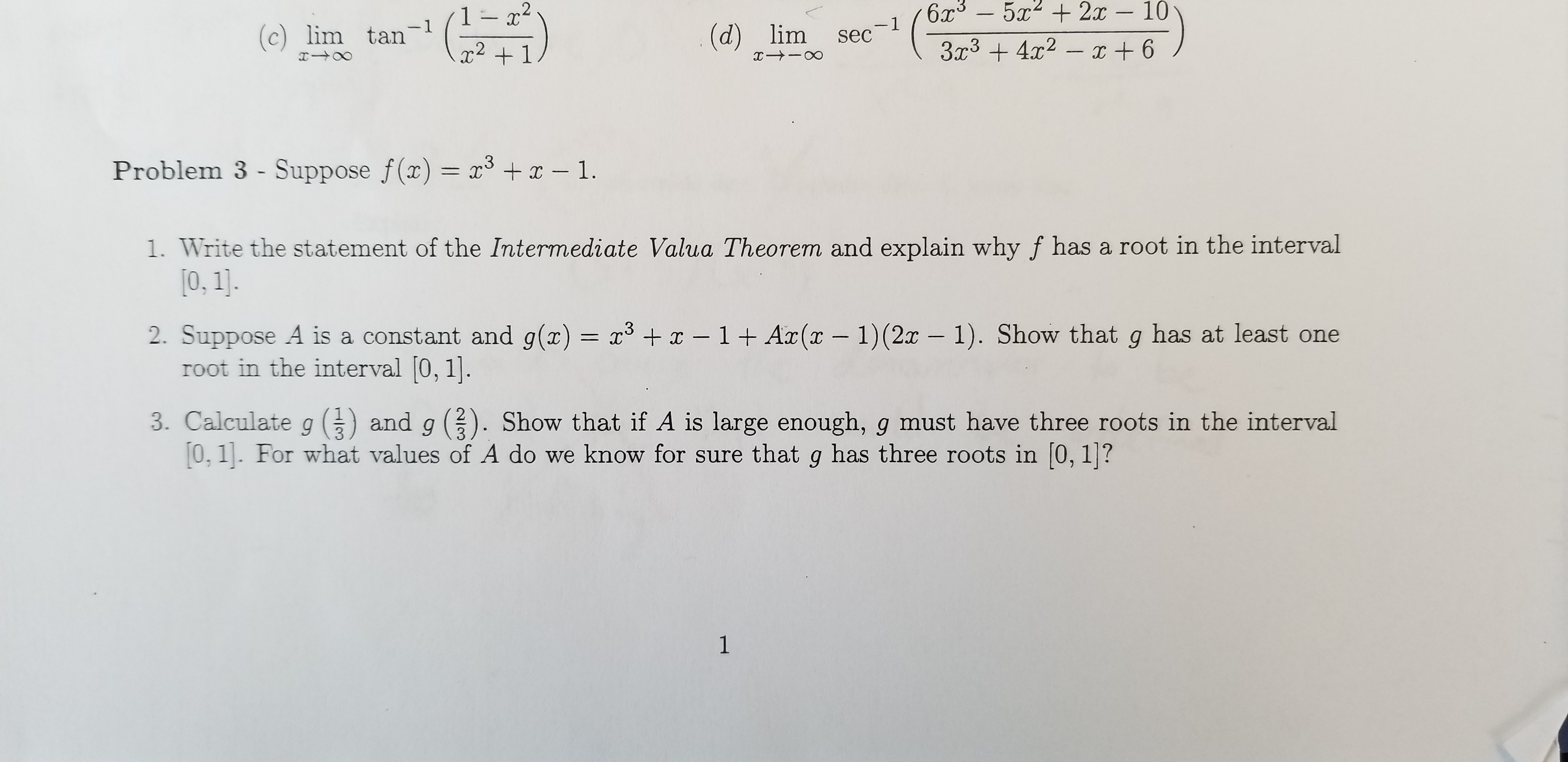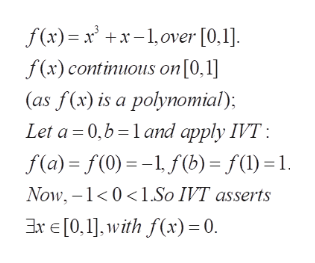# 6x3-5 2x - 103r342 61- 2-1sec-1(c) lim tan(d) lim2Problem 3 - Suppose f(x) = x x -1.31. Write the statement of the Intermediate Valua Theorem and explain why f has a root in the interval0, 1].2. Suppose A is a constant and g(z) = x3in the interval 0, 1].x -1 + Ar(x - 1) (2x - 1). Show that g has at least oneand g 33. Calculate g0, 1. For what values of A do we know for sure that g has three roots in [0, 1|?Show that if A is large enough, g must have three roots in the intervalC31

Question
5 views

Problem 3 in the image Parts 1, 2 , and 3help_outlineImage Transcriptionclose6x3-5 2x - 10 3r342 6 1- 2 -1 sec -1 (c) lim tan (d) lim 2 Problem 3 - Suppose f(x) = x x -1. 3 1. Write the statement of the Intermediate Valua Theorem and explain why f has a root in the interval 0, 1]. 2. Suppose A is a constant and g(z) = x3 in the interval 0, 1]. x -1 + Ar(x - 1) (2x - 1). Show that g has at least one and g 3 3. Calculate g 0, 1. For what values of A do we know for sure that g has three roots in [0, 1|? Show that if A is large enough, g must have three roots in the interval C3 1 fullscreen
check_circle

Step 1

The problems are concerned with the application of Intermediate value theorems in different contexts

Step 2

a) Intermediate value theroem: Let f(x) be a continuous function defined on the interval [a ,b]. Then f(x) assumes all the values between f(a) and f(b). In other words, if y is between f(a) and f(b) , then there exists an x in [a,b] with f(x)=y

Step 3

(a) Applying IVT (intermediate value theorem), we see that f(x)=...help_outlineImage Transcriptionclosef(x) xxover [0,1] f(x) continuous on[0,1] (as f(x) is a polynomial) Let a 0, b 1 and apply IVT f(a) f(0)- f (b) = f()-1 Now,-1 01.So IVT asserts ExE0,],with f(x) = 0. fullscreen

### Want to see the full answer?

See Solution

#### Want to see this answer and more?

Solutions are written by subject experts who are available 24/7. Questions are typically answered within 1 hour.*

See Solution
*Response times may vary by subject and question.
Tagged in

### Continuity